﻿ 春光油田储层砂岩地震岩石物理模型构建及其应用 The Establishment of Seismic Rock Physics Model of Reservoir Sandstone in Chunguang Oilfield and Its Application

Journal of Oil and Gas Technology
Vol.40 No.05(2018), Article ID:27420,11 pages
10.12677/JOGT.2018.405101

The Establishment of Seismic Rock Physics Model of Reservoir Sandstone in Chunguang Oilfield and Its Application

Xue Xiao, Zhenfeng Yang, Chunfeng Yang, Pengfei Wang, Feng Li

Research Institute of Exploration and Development, Henan Oilfield Company, SINOPEC, Zhengzhou Henan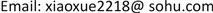Received: Sep. 12th, 2017; accepted: Jul. 21st, 2018; published: Oct. 15th, 2018ABSTRACT

Seismic rock physics model was an important tool for analyzing the rules of seismic elastic variation of reservoir rock and the prediction of hydrocarbon. The existing methods seldom considered the complex pore structure characteristics of reservoir rock when the rock physical model was established, which resulted in some errors in the prediction results. Systematical rock physics experiments were conducted on the sandstone samples of Shawan Formation of Chunguang Oilfield in Chepaizi Area of Xinjiang Province. After determining the pore system and concentration, the non-interaction approximation (NIA) was used to analyze the effects of porosity, pore shape and the concentration of micro-crack to the elastic properties of the dry frame of sandstone samples. The test results were used for calibrating the model parameters. Furthermore, a rock physical model was established to characterize the seismic elasticity of the rock skeleton of the target reservoir. The results of the model were in good agreement with the experimental results. According to the experimental results and model results, the jet flow model is used to replace the traditional Gassmann Equation for fluid replacement, which can better explain the ultrasonic frequency test results of fluid saturated sandstone samples.

Keywords:Pore Structure, Rock Physical Modeling, Non-interaction Approximation, Squirting-fluid ModelCopyright © 2018 by authors, Yangtze University and Hans Publishers Inc.

This work is licensed under the Creative Commons Attribution International License (CC BY).

http://creativecommons.org/licenses/by/4.0/

1. 引言

2. 试验样品制备与测量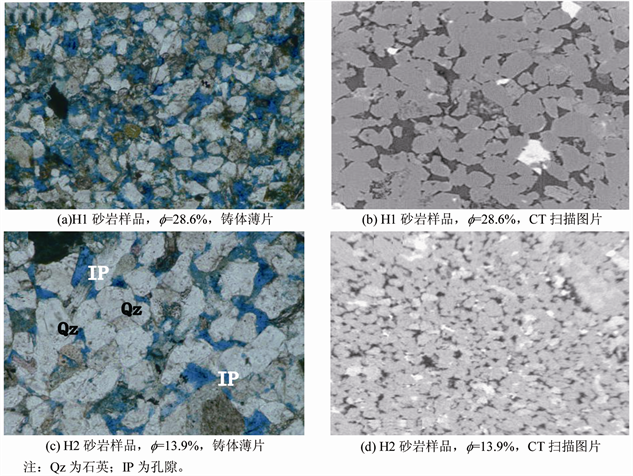Figure 1. The characteristics of micro-pore structures of reservoir sandstone samples

3. 样品地震岩石物理特征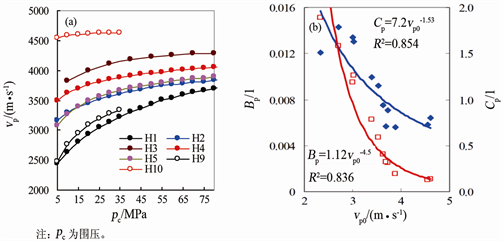Figure 2. The variation of vp with confining pressure under dry condition of sample (a) and relationship between P-wave fitting Factor and vp (b)

${p}_{\text{close}}=\left[\frac{\text{π}{E}_{\text{s}}}{4\left(1-{\upsilon }_{\text{s}}^{2}\right)}\right]\alpha \approx {E}_{\text{s}}\alpha$ (1)

$\begin{array}{l}{v}_{\text{pd}}\left({p}_{\text{e}}\right)=-{C}_{\text{p}}\mathrm{exp}\left(-d\cdot {p}_{\text{e}}\right)+{v}_{\text{p0}}+{B}_{\text{p}}{p}_{\text{e}}\\ {v}_{\text{sd}}\left({p}_{\text{e}}\right)=-{C}_{\text{s}}\mathrm{exp}\left(-d\cdot {p}_{\text{e}}\right)+{v}_{\text{s0}}+{B}_{\text{s}}{p}_{\text{e}}\end{array}$ (2)

4. 岩石物理模型

4.1. 岩石骨架模型

4.1.1. 模型建立

$S={S}_{0}+\Delta {S}_{\text{crack}}+\Delta {S}_{\text{stiff}}$ (3)

$\begin{array}{l}{K}_{\text{stiff}}={K}_{0}{\left(1+\frac{p{\varphi }_{\text{t}}}{1-{\varphi }_{\text{s}}}\right)}^{-1}\\ {G}_{\text{stiff}}={G}_{0}{\left(1+\frac{q{\varphi }_{\text{t}}}{1-{\varphi }_{\text{s}}}\right)}^{-1}\end{array}$ (4)

$\begin{array}{l}{K}_{\text{d}}={K}_{\text{stiff}}{\left(1+\frac{P{\varphi }_{\text{c}}}{1-{\varphi }_{\text{c}}}\right)}^{-1}\\ {G}_{\text{d}}={G}_{\text{stiff}}{\left(1+\frac{Q{\varphi }_{\text{c}}}{1-{\varphi }_{\text{c}}}\right)}^{-1}\end{array}$ (5)

$\epsilon =\frac{3}{4\text{π}\alpha }{\varphi }_{\text{c}}$ (6)

$\begin{array}{l}{K}_{\text{d}}={K}_{\text{stiff}}{\left(1+\frac{16\left(1-{\upsilon }_{\text{stiff}}^{2}\right)}{9\left(1-2{\upsilon }_{\text{stiff}}\right)}\epsilon \right)}^{-1}\\ {G}_{\text{d}}={G}_{\text{stiff}}{\left(1+\frac{32\left(1-{\upsilon }_{\text{stiff}}\right)\left(5-{\upsilon }_{\text{stiff}}\right)}{45\left(2-{\upsilon }_{\text{stiff}}\right)}\epsilon \right)}^{-1}\end{array}$ (7)

4.1.2. 模型试验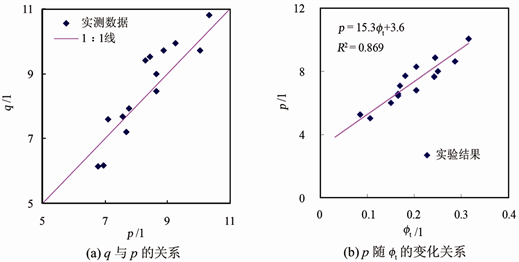Figure 3. The relationship of pore shape factor of sandstone samples in Shawan Formation of Chunguang oilfieldFigure 4. The feature of pc Change with ft and the relationship between ft, fc and ε0

$\epsilon \left({p}_{\text{e}}\right)={\epsilon }_{0}\mathrm{exp}\left(-d\cdot {p}_{\text{e}}\right)$ (8)

$\begin{array}{l}{K}_{\text{d}}={K}_{0}{\left[1+p\cdot {\varphi }_{\text{t}}+2.46\cdot {\epsilon }_{0}\mathrm{exp}\left(-d\cdot {p}_{\text{e}}\right)\right]}^{-1}\\ {G}_{\text{d}}={G}_{0}{\left[1+q\cdot {\varphi }_{\text{t}}+1.59\cdot {\epsilon }_{0}\mathrm{exp}\left(-d\cdot {p}_{\text{e}}\right)\right]}^{-1}\end{array}$ (9)Figure 5. The comparison of measured vp, vs and theoretically calculated results under different pressures

4.2. 孔隙流体作用

$\begin{array}{l}\frac{1}{{K}_{\text{mf}}\left({p}_{\text{e}},\omega \right)}=\frac{1}{{K}_{\text{h}}}+\frac{1}{\frac{1}{\frac{1}{{K}_{\text{d}}\left({p}_{\text{e}}\right)}-\frac{1}{{K}_{\text{h}}}}+\frac{3i\omega \eta }{8{\varphi }_{\text{c}}{\alpha }_{\text{c}}^{2}}}\\ \frac{1}{{G}_{\text{mf}}\left({p}_{\text{e}},\omega \right)}=\frac{1}{{G}_{\text{d}}\left({p}_{\text{e}}\right)}-\frac{4}{15}\left(\frac{1}{{K}_{\text{d}}\left({p}_{\text{e}}\right)}-\frac{1}{{K}_{\text{mf}}\left({p}_{\text{e}},\omega \right)}\right)\end{array}$ (10)

$\begin{array}{l}\frac{1}{{K}_{\text{sat}}\left({p}_{\text{e}},\omega \right)}=\frac{1}{{K}_{0}}+\frac{{\varphi }_{\text{s}}\left(\frac{1}{{K}_{\text{f}}}-\frac{1}{{K}_{0}}\right)}{1+{\varphi }_{\text{s}}\left(\frac{1}{{K}_{\text{f}}}-\frac{1}{{K}_{0}}\right)/\left(\frac{1}{{K}_{\text{mf}}\left({p}_{\text{e}},\omega \right)}-\frac{1}{{K}_{0}}\right)}\\ {G}_{\text{sat}}\left({p}_{\text{e}},\omega \right)={G}_{\text{mf}}\left({p}_{\text{e}},\omega \right)\end{array}$ (11)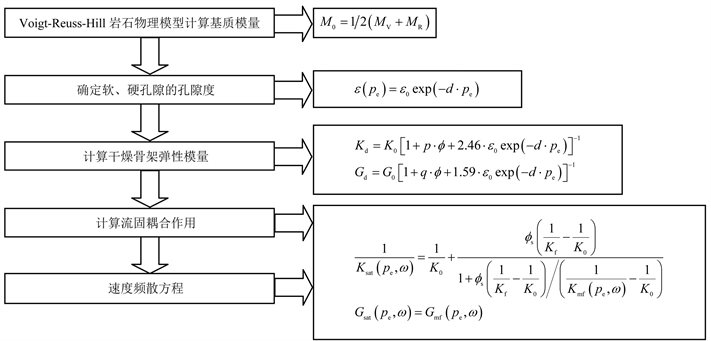注：M0为岩石弹性模量，MPa；MV为利用Voigt公式计算得到的岩石弹性模量上限，MPa；MR为利用Reuss公式计算得到的岩石弹性模量下限，MPa。

Figure 6. The procedures of seismic rock physics modeling based on porosity and pore shape

5. 结语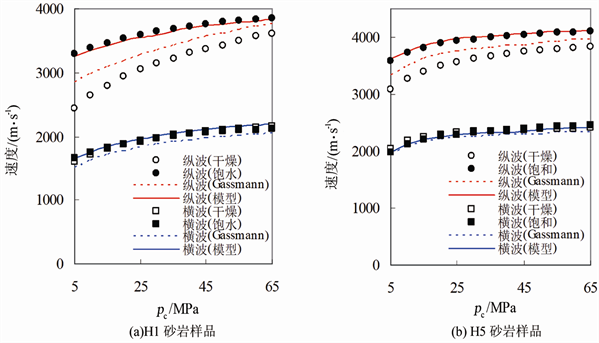Figure 7. The interpretation of ultrasonic experimental results

 Avseth, P., Mukerji, T. and Mavko, G. (2005) Quantitative Seismic Interpretation. Cambridge University Press, Cambridge, 48-108. https://doi.org/10.1017/CBO9780511600074

 魏嘉. 定量地震解释离我们有多远[J]. 勘探地球物理进展, 2006, 29(6): 433-437.

 Tang, J.W. (2008) Discussion on Several Issues about Seismic Rock Physics. Geophysical Prospecting for Petroleum, 47, 398-404.

 邓继新, 王尚旭, 伍向阳. 东营凹陷储层砂岩的地震特征及对岩石结构的指示作用[J]. 石油与天然气地质, 2009, 30(3): 294-299.

 Yin, X.Y., Zong, Z.Y. and Wu, G.C. (2015) Research on Seismic Fluid Identification Driven by Rock Physics. Science China (Earth Sciences), 58, 159-171. https://doi.org/10.1007/s11430-014-4992-3

 Mavko, G., Mukerji, J. and Dvorkin, J. (2003) The Rock Physics Handbook: Tools for Seismic Analysis in Porous Media. Cambridge University Press, New York, 1-329.

 Han, D.H., Nur, A. and Morgan, D. (1986) Effect of Porosity and Clay Content on Wave Velocities in Sandstones. Geophysics, 51, 2093-2107. https://doi.org/10.1190/1.1442062

 Xu, S. and White, R.E. (1995) A New Velocity Model for Clay-Sand Mixtures. Geophysical Prospecting, 43, 91-118. https://doi.org/10.1111/j.1365-2478.1995.tb00126.x

 Gassmann, F. (1951) Elastic Waves through a Packing of Spheres. Geophysics, 16, 673-685. https://doi.org/10.1190/1.1437718

 邓继新, 王尚旭. 基于统计岩石物理的含气储层饱和度与孔隙度联合反演[J]. 石油天然气学报(江汉石油学院学报), 2009, 31(1): 48-52.

 Batzle, M.L., Han, D. and Hofmann, R. (2006) Fluid Mobility and Frequency-Dependent Seismic Velocity-Direct Measurements. Geophysics, 71, 1-9. https://doi.org/10.1190/1.2159053

 Müller, T.B., Gurevich, B. and Lebedev, M. (2010) Seismic Wave Attenuation and Dispersion Resulting from Wave-Induced Flow in Porous Rocks: A Review. Geophysics, 75, 147-164. https://doi.org/10.1190/1.3463417

 Pride, S.R., Berryman, J.G. and Harris, J.M. (2004) Seismic Attenuation due to Wave-Induced Flow. Journal of Geophysical Research, 109, B01201. https://doi.org/10.1029/2003JB002639

 邓继新, 王尚旭, 俞军. 频散作用对储层砂岩速度实验结果的影响分析[J]. 石油物探, 2005, 44(4): 334-338.

 Deng, J.X., Zhou, H. and Wang, H. (2015) The Influence of Pore Structure in the Reservoir Sandstone on the Dispersion Properties of Elastic Wave. Chinese Journal of Geophysical Research (in Chinese), 58, 3389-3400.

 Walsh, J.B. (1965) The Effect of Cracks on the Compressibility of Rocks. Journal of Geophysical Research, 70, 381-389. https://doi.org/10.1029/JZ070i002p00381

 Vernik, L. and Kachanov, M. (2010) Modeling Elastic Properties of Siliciclastic Rocks. Geophysics, 75, 171-182. https://doi.org/10.1190/1.3494031

 Mori, T. and Tanaka, K. (1973) Average Stress in Matrix and Average Elastic Energy of Materials with Misfitting Inclusions. Acta Materialia, 21, 571-574. https://doi.org/10.1016/0001-6160(73)90064-3

 Gurevich, B., Makarynska, D., Paula, O., et al. (2010) A Simple Model for Squirt-Flow Dispersion and Attenuation in Fluid-Saturated Granular Rocks. Geophysics, 75, 109-120. https://doi.org/10.1190/1.3509782

[编辑] 龚丹